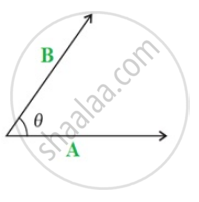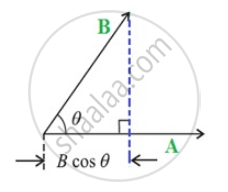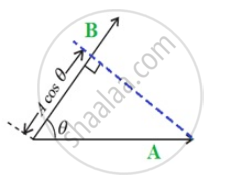Department of Pre-University Education, KarnatakaPUC Karnataka Science Class 11

# Introduction of Work, Energy and Power

• The Scalar Product

## Notes

#### Introduction

We use the term ‘work’ in everyday conversation to mean many different things. We talk about going to work, doing homework, working in class. Physicists mean something very specific when they talk about work.
In Physics we use the term work to describe the process of transferring energy from object or system to another or converting energy from one form to another.
You will learn that work and energy are closely related to Newton's laws of motion. You shall see that the energy of an object is its capacity to do work and doing work is the process of transferring energy from one object or form to another by means of a force.
In other words,

• an object with lots of energy can do lots of work.

• when object A transfers energy to object B, the energy of object A decreases by the same amount as the energy of object B increases, we say that object A does work on object B.

Lifting objects or throwing them requires that you do work on them. Even making an electrical current flow requires that something do work. Objects or systems must have energy to be able to do work on other objects or systems by transferring some of their energy.
We use the words work, energy and power in our day-to-day life often.However, their meaning differ from the meaning we get from scientific definitions.
In physics, work is defined as a force causing the movement or displacement of an object.
Power is the rate of doing work or of transferring heat, i.e. the amount of energy transferred or converted per unit time
Energy is the ability to do work

#### Scalar product:

“The scalar product or dot product of any two vectors A and B, denoted as A.B (Read A dot B) is defined as,

A · B = |A| |B| cos θ

where θ is the angle between the two vectors."

• A, B and cos θ are scalars, the dot product of A and B is a scalar quantity. Both vectors, A and B, have a direction but their scalar product does not have a direction.

• B is the product of the magnitude of A and the component of B along A. Alternatively, it is the product of the magnitude of B and the component of A along B.• B = B.A, i.e. Scalar product is commutative.

• (B + C) = A.B + A.C, i.e. Scalar product is distributive.

• Further, λ A. (λ B) = λ (A.B) where λ is a real number.

If you would like to contribute notes or other learning material, please submit them using the button below.